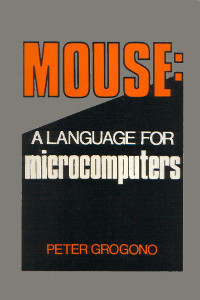Thursday, 23 August 2012

Mouse: a Language for Microcomputers by Peter GrogonoMouse is a stack-based interpreted programming language descended from MUSYS, an earlier language for a DEC PDP/8 with 4096 words of memory.

In Mouse: a Language for Microcomputers, Grogono defines the language and develops an intepreter step-by-step as he introduces each feature of the language.

The first few chapters cover the basics: postfix expressions, variables, control structures, pointers and macros. Later chapters include two complete Mouse interpreters, one in Pascal, the other in Z80 assembly language.

Here's a brief summary of the Mouse language. Instructions pop their operands from and push their result on the stack. ( before -- after ) shows the stack effects of each operation.

Maths / Logic

Mouse is looking pretty Forthlike so far! \ is the equivalent of MOD in Forth:

• + - ( x y -- z ) calculate z, the sum of x+y
• - - ( x y -- z ) calculate z, the difference of x-y
• * - ( x y -- z ) calculate z, the product of x×y
• / - ( x y -- z ) calculate z, the quotient of x/y
• \ - ( x y -- z ) calculate z, the remainder of x/y
• < - ( x y -- z ) if x<y then z is true, otherwise false
• = - ( x y -- z ) if x=y then z is true, otherwise false
• > - ( x y -- z ) if x>y then z is true, otherwise false

Input / Output

Only ? doesn't have an exact equivalent in Forth. ?' is the same as Forth's KEY. !' is the same as EMIT and ! is .:

• ? - ( -- x ) read a number x from the keyboard
• ?' - ( -- x ) read a character x from the keyboard
• ! - ( x -- ) display a number x
• !' - ( x -- ) display a character x
• "…" - ( -- ) display the quoted string

Peek and Poke

: is the equivalent of ! in Forth. . is the equivalent of @:

• : - ( x addr -- ) store x in address addr

Control Structures

[ … | … ] is similar to Forth's IF … ELSE … THEN.

( … ↑ … ) is similar to Forth's BEGIN … WHILE … REPEAT:

• [ - ( x -- ) if x is false, jump to the matching | or ]
• | - ( -- ) jump to the matching ] (not always implemented)
• ] - ( -- ) end a [ … | … ] structure
• ( - ( -- ) start a loop
• - ( x -- ) exit loop if x is false (often rendered as ^)
• ) - ( -- ) end loop, jump back to matching (
• ~ - ( -- ) the remainder of the line is a comment

Macro Definitions

• #x; - ( -- ) call macro x
• \$x - ( -- ) define macro x
• @ - ( -- ) end macro definition
• % - ( x -- z ) access macro parameter

Macros are the Mouse equivalent of subroutines. A macro is defined by \$x … @ and called with #x;. Parameters can be passed between the macro name and semicolon. For example #x,7,5,9; will pass the parameters 7, 5 and 9 to x.

A macro accesses it's parameters using %: 1% for the first, 2% for the second, etc. A parameter is evaluated every time it's accessed and can be almost any valid Mouse code.

A macro has 26 local variables, A to Z. 26 macro names are available, A to Z.

Example Code

Here are a few classic examples:

• Hello World (which recently celebrated it's 40th birthday)
• Fibonacci Numbers (the typical bad example of recursion)
• Greatest Common Divisor (a better example of recursion)

Hello, World

"Hello, World!"\$

Displays the string Hello, World. The exclamation mark isn't printed. An exclamation in a string instructs the interpreter to print a line break. All Mouse programs end with \$.

Fibonacci Numbers

\$F
1% N:                   ~ store parameter in N
N. 2 < [ N. ]           ~ if N < 2 then return N
N. 1 > [ #F, N. 1 - ;   ~ otherwise calculate F(N-1)
#F, N. 2 - ;   ~  | and F(N-2)
+ ]            ~  | and return their sum
@

F calculates Fibonacci numbers using the recurrence relation Fn = Fn-1 + Fn-2 with F0 = 0, F1 =1. Note this is really slow, Ο(φn). Calculating F40 takes 6 minutes. There are better ways to calculate Fibonacci numbers!

Greatest Common Divisor

\$G
1% X: 2% Y:                     ~ store parameters
X. Y. = [ X. ]                  ~ if X = Y then GCD = X
X. Y. > [ #G, X. Y. - , Y. ; ]  ~ otherwise subtract the
X. Y. < [ #G, Y. X. - , X. ; ]  ~  | smallest from the
@                               ~  | largest and recurse

Another example of recursion. G calculates the GCD using Dijkstra's method.

• Grogono, Peter Mouse: A Language for Microcomputers.
New York: Petrocelli Books, 1983.
• David G Simpson has published several interpreters for Mouse.

1.Having something like

+ - ( x y -- z ) means that this is a postfix expression. They are sometimes not that easy to decipher. Most languages out there use infix expressions (such as x + y - z). LISP uses postfix (where you have something like (+ x y))

Thanks for sharing...

1.I think you meant LISP is PREfix.

2.This is a stack based language, therefore postfix/RPN should be expected.

2.I would rather use a professional programming environment, made for professionals to create reliable fast professional code.

You should use Visual Basic.

1.Microsoft shill? :/

2.Ha ha, good joke...